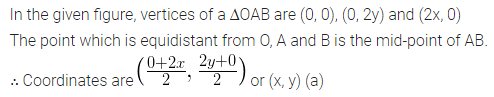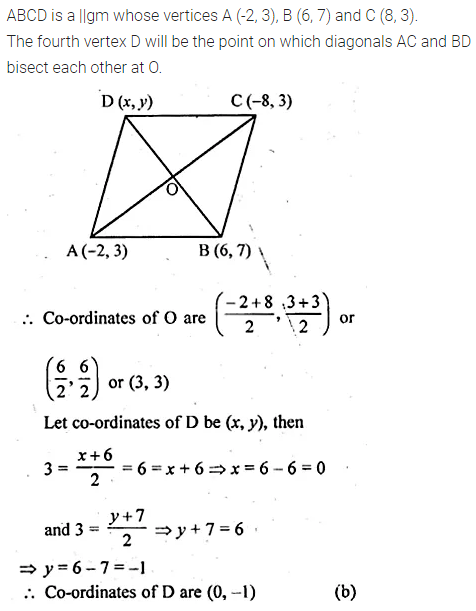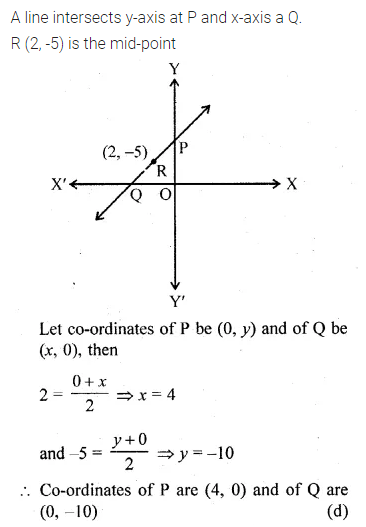# ML Aggarwal Class 10 Solutions for ICSE Maths Chapter 11 Section Formula MCQS

## ML Aggarwal Class 10 Solutions for ICSE Maths Chapter 11 Section Formula MCQS

ML Aggarwal Class 10 Solutions for ICSE Maths Chapter 11 Section Formula MCQS

Choose the correct answer from the given four options (1 to 12) :

Question 1.
The points A (9, 0), B (9, 6), C (-9, 6) and D (-9, 0) are the vertices of a
(a) rectangle
(b) square
(c) rhombus
(d) trapezium
Solution:Question 2.
The mid-point of the line segment joining the points A (-2, 8) and B (-6, -4) is
(a) (-4, -6)
(b) (2, 6)
(c) (-4, 2)
(d) (4, 2)
Solution:Question 3.
If $$P\left( \frac { a }{ 3 } ,4 \right)$$ segment joining the points Q (-6, 5) and R (-2, 3), then the value of a is
(a) -4
(b) -6
(c) 12
(d) -12
Solution:Question 4.
If the end points of a diameter of a circle are A (-2, 3) and B (4, -5), then the coordinates of its centre are
(a) (2, -2)
(b) (1, -1)
(c) (-1, 1)
(d) (-2, 2)
Solution:Question 5.
If one end of a diameter of a circle is (2, 3) and the centre is (-2, 5), then the other end is
(a) (-6, 7)
(b) (6, -7)
(c) (0, 8)
(d) (0, 4)
Solution:Question 6.
If the mid-point of the line segment joining the points P (a, b – 2) and Q (-2, 4) is R (2, -3), then the values of a and b are
(a) a = 4, b = -5
(b) a = 6, b = 8
(c) a = 6, b = -8
(d) a = -6, b = 8
Solution:Question 7.
The point which lies on the perpendicular bisector of the line segment joining the points A (-2, -5) and B (2, 5) is
(a) (0, 0)
(b) (0, 2)
(c) (2, 0)
(d) (-2, 0)
Solution:Question 8.
The coordinates of the point which is equidistant from the three vertices of ∆AOB (shown in the given figure) are
(a) (x, y)
(b) (y, x)
(c) $$\left( \frac { x }{ 2 } ,\frac { y }{ 2 } \right)$$
(d) $$\left( \frac { y }{ 2 } ,\frac { x }{ 2 } \right)$$Solution:Question 9.
The fourth vertex D of a parallelogram ABCD whose three vertices are A (-2, 3), B (6, 7) and C (8, 3) is
(a) (0, 1)
(b) (0, -1)
(c) (-1, 0)
(d) (1, 0)
Solution:Question 10.
A line intersects the y-axis and x-axis at the points P and Q respectively. If (2, -5) is the mid-point of PQ, then the coordinates of P and Q are, respectively
(a) (0, -5) and (2, 0)
(b) (0, 10) and (-4, 0)
(c) (0, 4) and (-10, 0)
(d) (0, -10) and (4, 0)
Solution:Question 11.
The points which divide the line segment joining the points (7, -6) and (3, 4) in the ratio 1 : 2 internally lies in the